# Venn Diagrams Questions for SSC CGL Set-2 PDF

0
880

## Venn Diagrams Questions for SSC CGL Set-2 PDF

Download SSC CGL Venn Diagrams Questions with answers set-2 PDF based on previous papers very useful for SSC CGL exams. Very important Vocabulary Words for SSC exams.

Question 1: Select the Venn diagram that best illustrates the relationship between the following classes.
Metal, Iron, Mercury

a)

b)

c)

d)

Question 2: In the following figure, rectangle represents Film directors, circle represents Cartoonists, triangle represents Campers and Square represents Cricketers. Which set of letters represents Cricketers who are also Cartoonists ?

a) IJ

b) CD

c) HG

d) DE

Question 3: Choose the Venn diagram from the given options which best represents the relationship amongst the following classes:
Cup, Table, Crockery

a)

b)

c)

d)

Question 4: Identify the diagram that best represents the relationship among the classes given below :
Doctors, Engineers, Lawyers

a)

b)

c)

d)

Question 5: Identify the diagram that best represents the relationship among the given classes.
Cricketer, Male, Actor

a)

b)

c)

d)

Question 6: Identify the diagram that best represents the relationship among the given classes.
Biology, Science, Subject

a)

b)

c)

d)

Question 7: Identify the diagram that best represents the relationship among the given classes.
Reptiles, Lizard, Lion

a)

b)

c)

d)

Question 8: Identify the diagram that best represents the relationship among the given classes.
Four wheelers, Jeep, Car

a)

b)

c)

d)

Question 9: Identify the diagram that best represents the relationship among the given classes.
Cap, Pencil, Apple

a)

b)

c)

d)

Question 10: Identify the diagram that best represents the relationship among the given classes.
Crockery, Cup, Plate

a)

b)

c)

d)

Iron is metal and mercury is also a metal so both of them fall under same category

Set of letters that represents Cricketers who are also Cartoonists = G + H

=> Ans – (C)

cup comes under crockery, table comes under furniture

All three entities are entirely different.

Hence, option D is the correct answer.

A male can be a cricketer or an actor, but an actor cannot be a cricketer.

here cricketer and actor defines field in which they work

for example sachin tendulkar acted in many ads but by profession he is a cricketer hence one can only be cricketer or an actor at one working profession

Thus, the venn diagram that best describes above relationship is :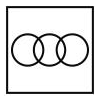=> Ans – (C)

Biology is a branch of science and science is a subject.

Thus, the venn diagram that best describes above relationship is :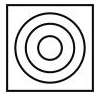=> Ans – (C)

All lizards comes under the category of lizards, but lions are mammals.

Thus, the venn diagram that best describes above relationship is :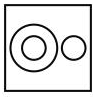=> Ans – (C)

Both jeep and car are different from each other, but comes under the category of four wheelers, hence the venn diagram that best describes above relation is :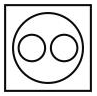=> Ans – (D)

Cap, pencil and apple are completely different from each other, hence do not intersect one another.

Thus, the venn diagram that best describes above relationship is :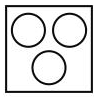=> Ans – (C)